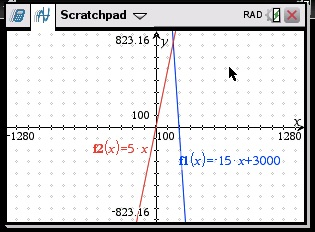Test 3 Section 4 #36

This is the calculator section; graphing will probably help you visualize what’s going on here. Don’t worry about the inequalities for now—just graph the lines. You’ll have to zoom out a bunch to see the intersection, but here’s what it looks like on my calculator:The inequalities tell you that y is less than or equal to both of those lines, and you’re looking for the greatest possible y-value in the solution set. In other words, you’re looking for the highest point that’s BELOW OR ON both lines. With the graph in front of you, it should be easier to see that the point you need has to be the intersection of the two lines. So use your calculator to find that:There you have it—the answer is 750.

Pro-tip: if you get another question like this, with two linear inequalities where you’re asked for the greatest (or least) possible value of one of the coordinates in the solution set, you’re looking for the intersection of the lines.

Once you know that, you may choose to just solve a problem like this algebraically next time:

–15x + 3000 = 5x
3000 = 20x
150 = x

If x = 150, at the intersection, then y ≤ 5(150); y ≤ 750.